Home » BASIC PROGRAMMING 3

# BASIC PROGRAMMING 3ARRAYS

A table is a data structure that enables data to be stored in the main memory of the computer. The term table is also known as array. An array variable is a data structure consisting of an ordered set of elements of the same data type. One advantage of arrays is that they allow for the logical grouping of data of the same type, for example the x and y coordinates of a particle.

Advantages of Storing Data in Tables

1. Each item of data does not have a unique variable name.
2. Program coding is reduced.
4. The homogeneous nature of data can be classified under one name.

One Dimensional Array

The smallest component of an array is called a cell or element and contains either a string literal or a number.

540 A cell containing an item of data

The cell are grouped together to form a one – dimensional table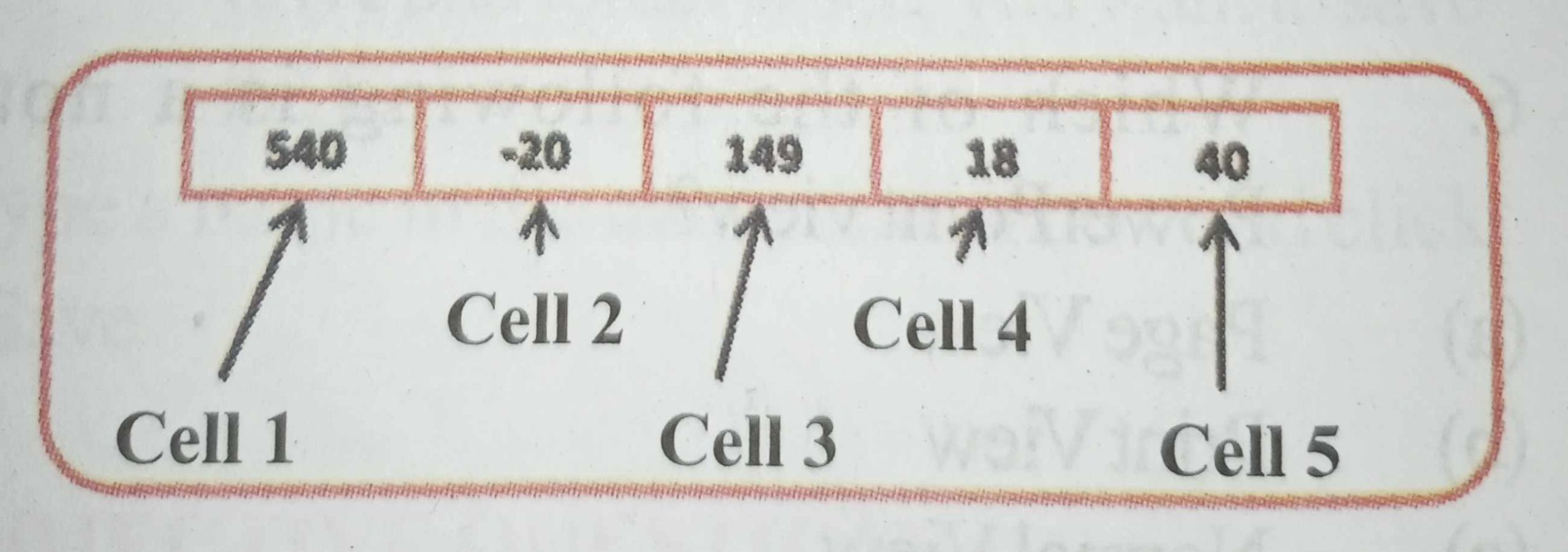A table can be given one data name, depending upon the type of data to be stored in the table using the same rules of composition as for data names.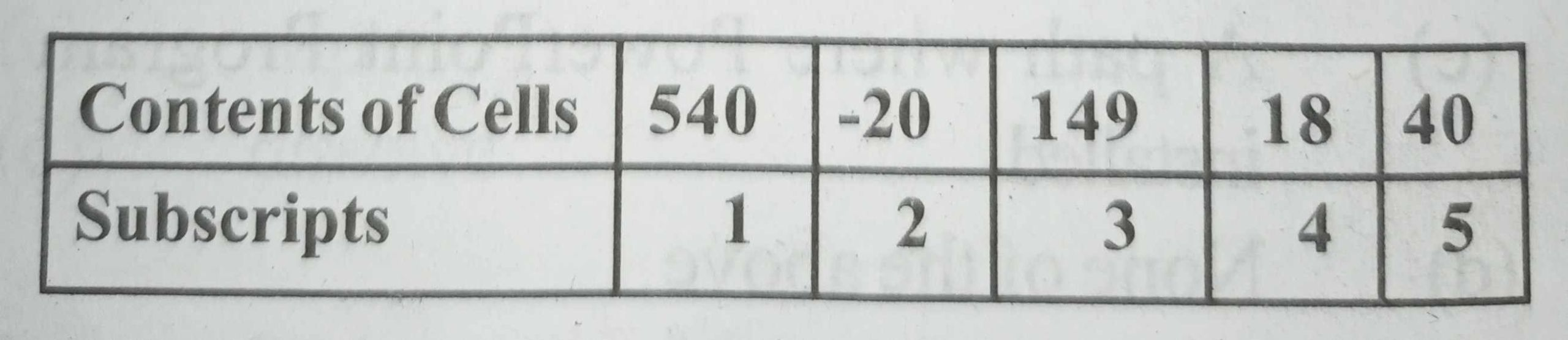Each cell is given an address called a subscript, that defines the position of a cell within a table. The contents of each cell is accessed by the name given to the table followed in parenthesis by the subscript of the cell.

Thus, A (1) = 540, A (2) = – 20, A (3) = 149, A (4) = 18, A (5) = 40.

The declaration of the name and size of the table is made using a DIM statement.

The declaration of array A is: 10 DIM A (4)

Where A is the name of table and (4) is the maximum number of cells required.

DIM Statement

Arrays are defined in a DIM statement and the total number of elements of an array is given in parentheses. An element of an array is specified by its subscript value. Arrays can be passed to a subroutine or a function, with empty parentheses and commas used to indicate dimension of the array.

DIMension Statement Format

The DIMension statement is used to describe subscripted variables and to define the length of strings.

DIMension Statement Form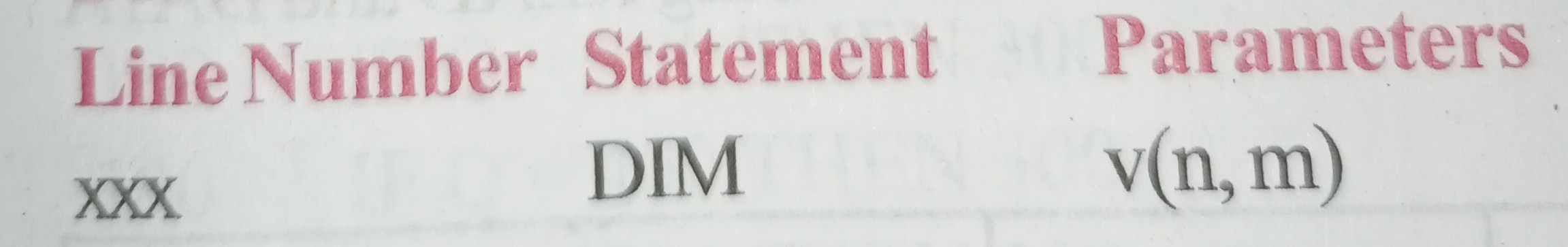where v is the name of the subscripted variable.

1) If the variable name (v) is numeric, and m is omitted, then n+1 is the number of elements in an array or vector.

Example 1

The following statement 10 DIM Q (6)

Describe seven numeric elements as follows: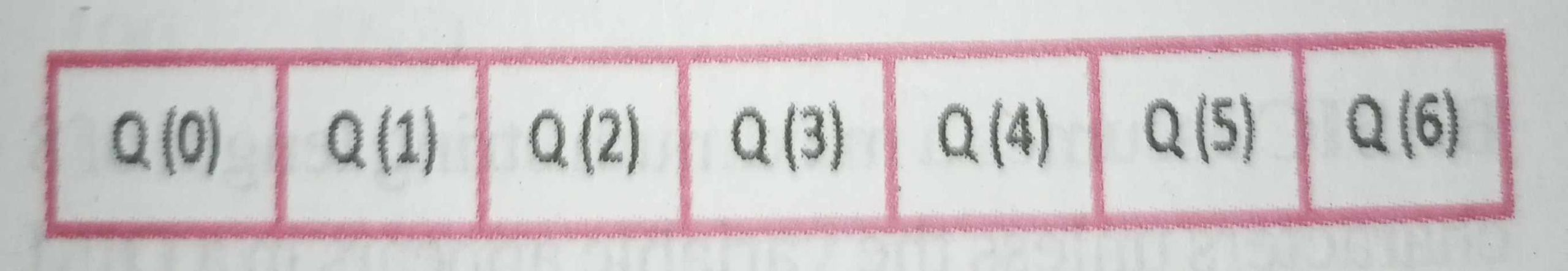To store a 5 in Q (4) and then display it, type:

20 LET Q (4) = 5

30 PRINT Q (4)

2) m is specified, then n + 1 is the number of rows in a two-dimensional array.

Example 2

The following statement

10 DIM Q (3,6)

Describes 28 numeric elements (4 x 7 =28) as follows:

Note: DIM A(m,n) = (n+1) (m+1) values.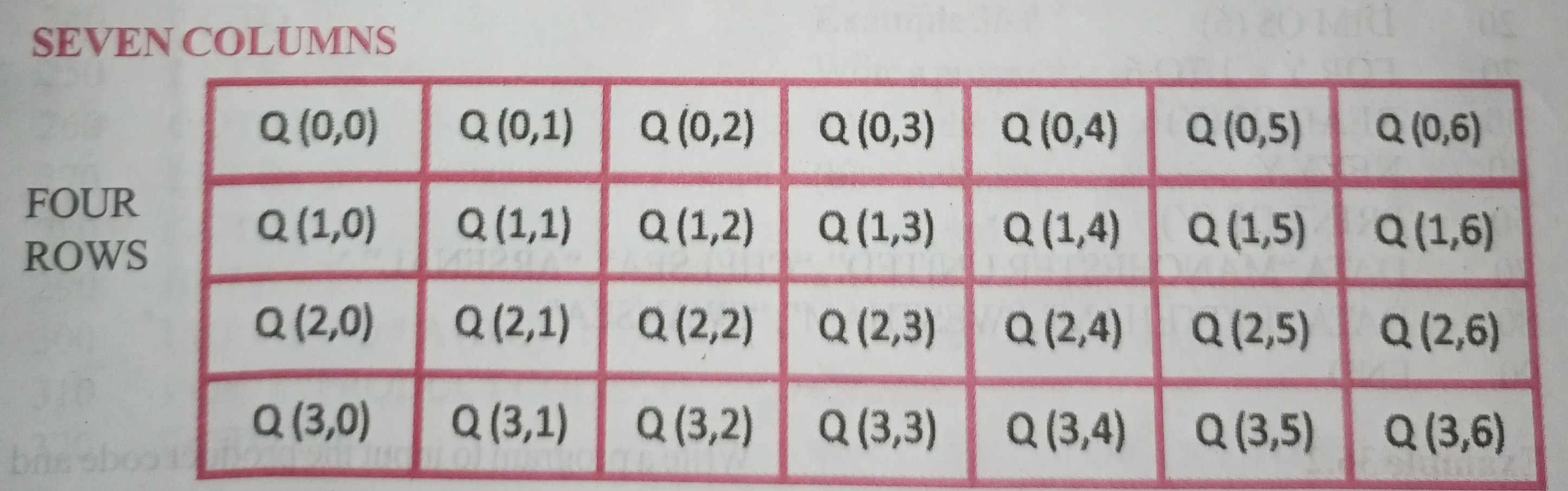3) if v is numeric, then m+1 is the number of columns in a two-dimensional array. If the variable name (v) is alphanumeric, and m is omitted, then n is the length of the string. This describes a single string, not a list, and cannot exceed 80.

m is specified, then n+1 is the number of strings in the list. This is a one dimensional array. if v is alphanumeric, then m is the length of each string in a one-dimensional list. It cannot exceed 80.

The parameters n and m must be integer constants. They are limited in size only by the amount of available memory. If a numeric variable is used in the program with a subscript but is not defined in a DIM statement, BASIC assigns it an array size of ten.

BASIC assumes a maximum string length of 8 characters unless the variable appears in a DIM statement. Two-dimensional string variables are not permitted.

When the variable is used in other statements, it is not permitted to have subscripts whose values are higher than those in the DIM statement. The first element of every array is automatically assumed to have a subscript of zero. Therefore, the number of boxes in a one dimensional array is n+ 1. The number of boxes in a two-dimensional array is (n+ 1)*(m+ 1). The first element in an array is v(0) or v(0,0). However, the “zero” elements can be disregarded in programming unless the user wishes to conserve memory. More than one array can be defined in a single DIM statement.

Example 1

Write a program to store the names of six football clubs in a table below using READ and DATA statement.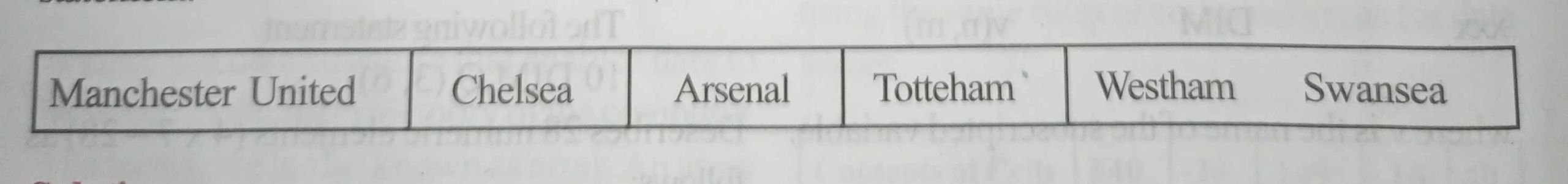Solution

10 REM DECLARING THE NAMES OF FOOTBALL CLUBS

20 DIM Q\$ (6)

30 FOR Y = 1 TO 6

50 NEXT Y

60 PRINT D\$ (Y)

70 DATA “MANCHESTER UNITED”, “CHELSEA”, “ARSENAL”,

80 DATA “TOTTEHAM”, “WESTHAM”, “SWANSEA”

90 END

Example 2

The prices of three different articles vary according to the quantities sold. The price bands are given in the table below: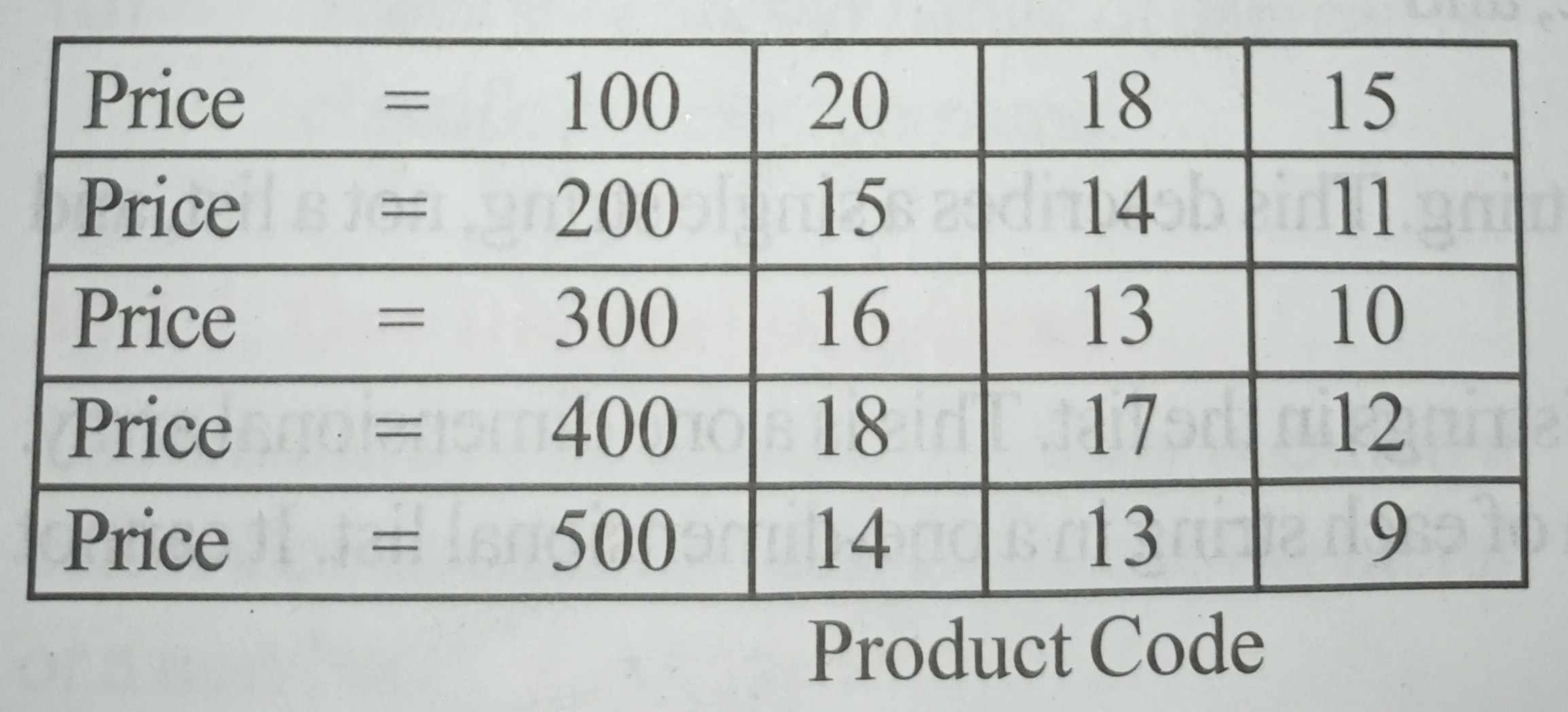Write a program to input the product code and quantity sold, calculate and print the cost of the sale. Terminate the program when the product code is out of range.

Solution

10 REM PROGRAM TO INPUT PRODUCT CODE AND PRINT COST OF SALE

20 DIM A(5,3)

30 FOR B = 1 TO 5

40 FOR C = 1 TO 3

60 NEXT C

70 NEXT B

80 DATA 20, 18, 15

90 DATA 15, 14, 11

100 DATA 16, 13, 10

110 DATA 18, 17, 12

120 DATA 14, 13, 9

130 INPUT “PRODUCT CODE”, P

140 IF P < 1 OR P > 3 THEN STOP

150 INPUT “QUANTITY SOLD”, Q

160 IF Q = 100 THEN 300

170 IF Q = 200 THEN 300

180 IF Q = 300 THEN 300

190 IF Q = 400 THEN 300

200 IF Q = 500 THEN 300

210 LET B = 5

220 GOTO

230 LET B = 4

240 GOTO

250 LET B = 3

260 GOTO

270 LET B = 2

280 GOTO

290 LET B= 1

300 LET = S = Q * A (B,C)

310 INPUT “PRODUCT CODE”, C

320 GOTO 140

Example 3

Write a program to calculate the volume of sphere with 12 different radii.

Solution

10 REM … “VOLUME OF SPHERE”

20 DEF FNX (R) = 1.3333*P*R^3

30 COUNT = 0

40 INPUT R

50 COUNT = COUNT + 1

60 LET P = 3.142

70 PRINT “VOLUME OF SPHERE WITH RADIUS, “R”, “IS” FXR(R)

80 PRINT

90 IF COUNT <> 12 THEN GO TO 40

100 END

Example 4

A two – dimensional table T, has 6 rows and 9 columns. Write a program to set the contents of every cell to 100.

Solution

10 DIM T(6, 9)

20 FOR I = 1 TO 6

30 FOR J = 1 TO 9

40 LET T(I, J) = 100

50 NEXT J

60 NEXT I

70 END

Example 5

Write a program to output:

• the odd integers between 1 and 49.
• the squares of even integers between 2 and 50.
• the sum of the cubes of odd integers between 11 and 49.

Solution

(5a)

10 FOR I = 1 TO 49

20 PRINT I

30 NEXT I

40 END

(5b)

10 FOR 1 = 2 TO 50

20 LET S = I*I

30 PRINT I, S

40 NEXT I

50 END

5(c)

10 LET S = 0

20 FOR 1 = 11 TO 49 STEP 2

30 LETS = (S+1)ˆ 3

40 NEXT I

50 PRINT “SUM OF CUBES”, S

60 END

Example 6

Write a program to print the arithmetic mean of a list of positive numbers. Terminate the procedure with zero.

Solution

10 REM …. PROGRAM TO CALCULATE ARITHMETIC MEAN

20 REM …. T TOTAL

30 REM ….N NUMBER OF NUMBERS

40 REM …. X CURRENT NUMBER

50 REM …. M ARITHMETIC MEAN

60 T = 0

70 N = 0

80 INPUT “NUMBER”, X

90 IF X = 0 THEN 140

100 T = T + X

110 N = N + 1

120 INPUT “NUMBER”, X

130 GO TO 90

140 M = T/N

150 PRINT “MEAN”, M

160 STOP

Example 7

Write a program to illustrate the coding of multiple selection using GO TO statement.

Solution

10 INPUT “CODE 1 -4”, C

20 INPUT “FIRST NUMBER”, N1

30 INPUT “SECOND NUMBER”, N2

40 ON C GOTO 50, 70, 90, 110

50 LET A = N1 + N2

60 GOTO 120

70 LET A = N1 – N2

80 GOTO 120

90 LET A = N1*N2

100 GOTO 120

110 LETA = N1/N2

130 STOP

Example 8

Repeat example 7 using IF …. THEN statement.

Solution

10 INPUT “CODE 1 -4”, C

20 INPUT “FIRST NUMBER”, N1

30 INPUT “SECOND NUMBER”, N2

40 IF C <> 1 THEN GOTO 70

50 LET A = N1 + N2

60 GOTO 140

70 IF C <> 2 THEN GOTO 100

80 LET A= N1 – N2

90 GOTO 140

100 IF C <> 3 THEN GOTO 130

110 LET A = N1*N2

120 GOTO 140

130 LET A = N1/N2

150 STOP

Example 9

Repeat example 7 using IF …. THEN … ELSE statement.

Solution

10 INPUT “CODE 1 -4”, C

20 INPUT “FIRST NUMBER”, N1

30 INPUT “SECOND NUMBER”, N2

40 IF C = 1 THEN LET A = N1 + N2

50 ELSE IF C = 2 THEN LET A = N1 – N2

60 ELSE IF C = 3 THEN LET A = N1*N2

70 ELSE LET A = N1/N2

90 STOP

Example 10

In an examination five pass grades and one fail grade is awarded:

A for marks between 80 and 100 inclusive.

B for marks between 70 and 79 inclusive

C for marks between 60 and 69 inclusive

D for marks between 50 and 59 inclusive

E for marks between 40 and 49 inclusive

F for marks below 40

Write a program to input an examination mark for a student and print the grade. Repeat the procedure until the examination mark is 850, then, print the total number of students in each of the six grades.

Solution

10 LET A = 0

20 LET B = 0

30 LET C = 0

40 LET D = 0

50 LET E = 0

60 LET F =0

70 INPUT “MARK”, M

80 WHILE <> 850

90 IF M < 80 THEN 130

100 LET A = A+1

120 GOTO 310

130 IF M < 70 THEN 170

140 LET B = B+1

160 GOTO 310

170 IF M < 60 THEN 210

180 LET C = C+1

200 GOTO 310

210 IF M < 50 THEN 250

220 LET D = D+1

240 GOTO 310

250 IF M < 40 THEN 290

260 LET E = E+1

280 GOTO 310

290 LET F = F+1

310 INPUT “MARK”, M

320 WEND

330 PRINT “A”, A

340 PRINT “B”, B

350 PRINT “C”, C

360 PRINT “D”, D

370 PRINT “E”, E

380 PRINT “F”, F

390 STOP

Example 11

Devise a program to store numbers in a two-dimensional array M having 4 rows and 5 columns and output the highest and lowest values in:

(a) Each row.

(b) Each column.

Solution

10 REM PROGRAM TO STORE NUMBERS IN TWO DIMENSIONAL ARRAY

20 DIM (4,5)

30 FOR I = 1 TO 4

40 FOR J = 1 TO 5

50 INPUT “ENTRY”, M(I, J)

60 NEXT J

70 NEXT I

80 REM SEARCH ROW FOR HIGHEST AND LOWEST VALUES

90 FOR I = 1 TO 4

100 B = M(I,1): S = M(I, 1)

110 FOR J = 2 TO 5

120 IF B < M(I,J) THEN B = M(I,J)

130 IF S > M(I,J) THEN S = M(I,J)

140 NEXT J

150 PRINT “ROW”;I; “MAX”; B; “MIN”;S

160 NEXT I

170 REM SEARCH COLUMN FOR HIGHEST AND LOWEST VALUES

180 FOR J = 1 TO 5

190 B = M(1,J): S = M(1,J)

200 FOR I = 2 TO 4

210 IF B < M(I,J) THEN B = M(I,J)

220 IF S > M(I,J) THEN S=M(I,J)

230 NEXT I

240 PRINT “COLUMN”;J; “MAX”; B; “MIN”;S

250 NEXT J

260 STOP

Example 12

A list of integers in the range 1 to 20 are defined in the following DATA statement.

DATA 16, 18, 1, 3, 20, 7, 5, 2,10, 19, 17, 13, 4, 6, 9, 11, 8, 14,12,15. Write a program to read the integers in a one – dimensional table containing 20 cells, using the integer value as subscript to the cell.

Solution

10 REM DEFINING ONE-DIMENSIONAL TABLE

20 DIM A(20)

30 FOR I = 1 TO 20

50 LET T(X) = X

60 NEXT I

70 DATA 16, 18, 1, 3, 20, 7, 5, 2,10, 19, 17, 13, 4, 6, 9, 11, 8, 14,12,15

80 FOR I = 1 TO 20

90 NEXT I

100 STOP

Example 13

Write a program to store ten names of people in a one – dimensional table and print the contents out.

Solution

10 DIM A\$ (10)

20 FOR I = 1 TO 10

30 INPUT A\$ (I)

40 PRINT A\$ (I)

50 NEXT I

60 STOP